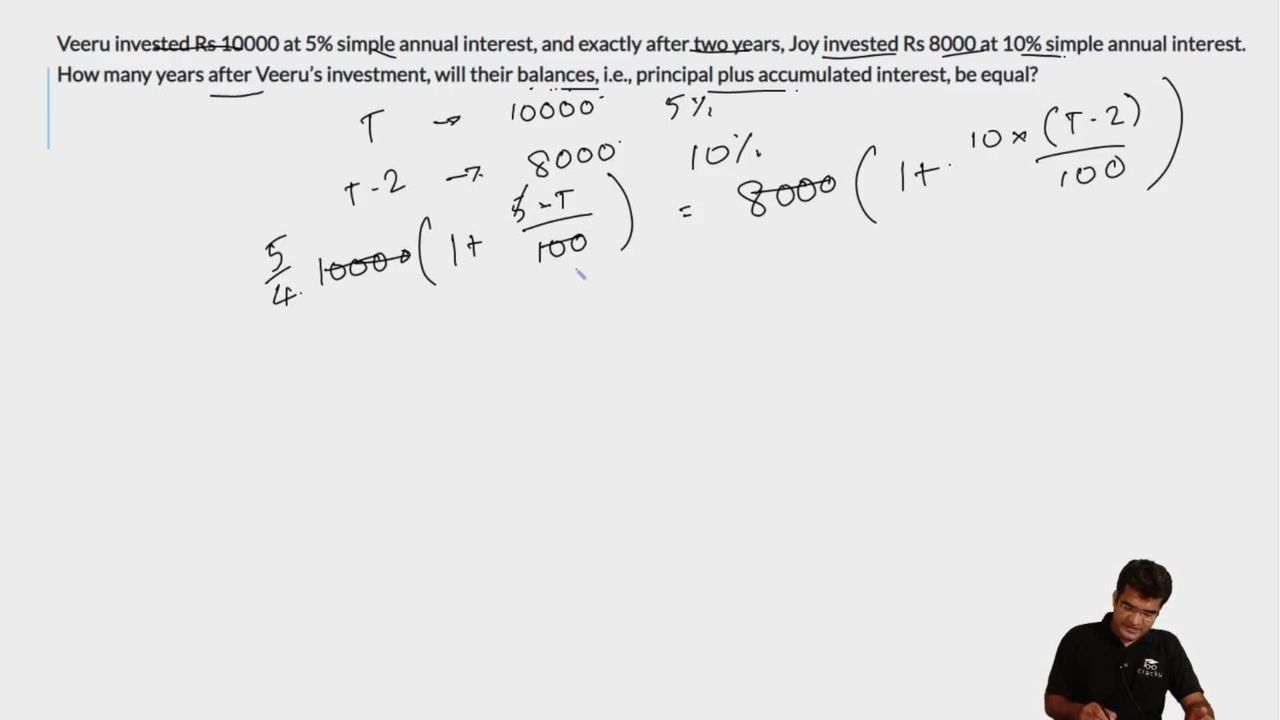Question 24

# Veeru invested Rs 10000 at 5% simple annual interest, and exactly after two years, Joy invested Rs 8000 at 10% simple annual interest. How many years after Veeru’s investment, will their balances, i.e., principal plus accumulated interest, be equal?

Solution

Let their individual Amounts be equal after 't' years. Let their initial investments amount to $$A_V$$ and $$A_J$$ ;

$$A_V\ =10,000\left(1+\frac{5t}{100}\right)$$ and $$A_J\ =8,000\left(1+\frac{10\left(t-2\right)}{100}\right)$$

Equating both: $$10,000\left(1+\frac{5t}{100}\right)\ =8,000\left(1+\frac{10\left(t-2\right)}{100}\right)$$

On simplifying both sides, we get: $$15t\ =\ 180\ ;\ t\ =\ 12$$

### View Video Solution